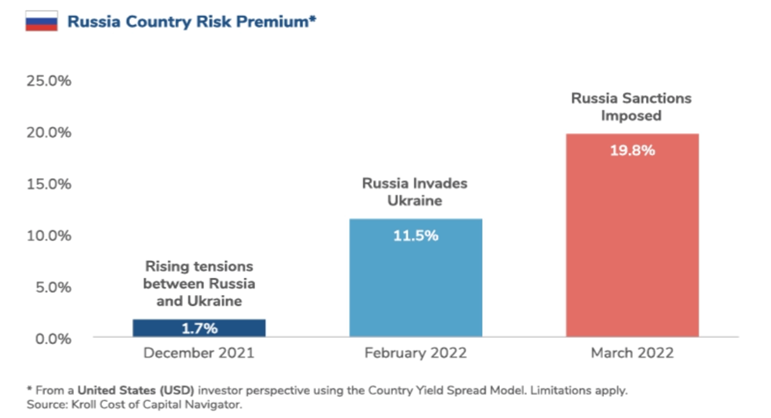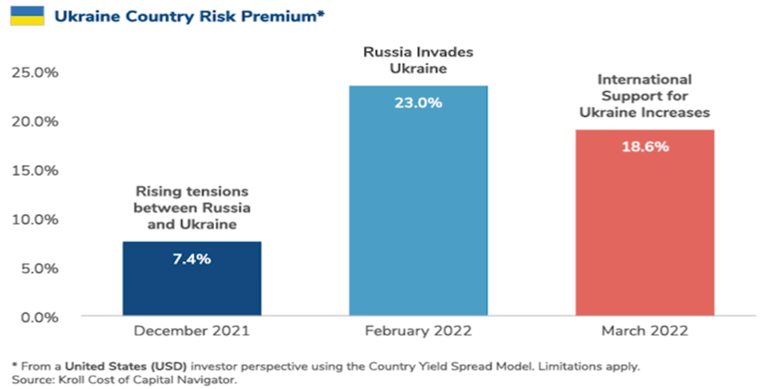Blog

Home / Blog

## Introduction to Country Risk Premium (CRP)?

Country Risk Premium (CRP) is the add on return asked by an investor to compensate them for the additional risk that when thinking of investing in a foreign country. When we compare operating or investing in the domestic market, the risk involved doesn’t change much. But when we cross borders, the riskiness may increase, sometimes very significantly.

Overseas investment opportunities are accompanied by a much greater risks & these risks makes an investor more sceptical, as a result, this spec of doubt makes the investor yearn for additional return over the risk-free benchmark (Usually taken as 10 bond yield on sovereign issued security). CRP is generally higher for developing economies than for developed economies.

## Country risk encompasses numerous factors, including:

• Political instability;
• Economic risks like recession or recession-like conditions, high inflation etc.;
• Sovereign debt burden and default probability;
• Currency fluctuations;
• Adverse government regulations (such as expropriation or currency controls).

## Estimation of Country Risk Premium:

There are two commonly used methods of estimating CRP:

• Sovereign Debt Method: CRP for a country can be computed by comparing the spread on sovereign debt yields between the target country and a much less-riskier and stable market, for example - U.S. or Germany.
• Equity Risk Method: When using this method of estimation, CRP is measured by comparing the relative volatility of equity market returns between the target country and a developed

## Country Risk Premium can be calculated through the below formula: *

CRP = Spread on Sovereign Bond Yield * (Annualized Standard Deviation on Equity Index / Annualized Standard Deviation on Bond Index)

Where:

Sovereign yield spread = Government bond yield denominated in a developed country currency − Treasury bond yield on a similar maturity bond in a developed country

There are three widely used approaches. The first one is based around default spread (country bonds or ratings), whereas the latter two use equity market volatility as an input in the estimating of country risk premium.

The most-simple and widely used proxy for country risk premium is the default spread on the country-issued bond. This default spread can be estimate in 3 different ways.

• Current Default spread on sovereign bonds or the (CDS Market): The default spread comes from either looking at the yields on bonds issued by the country in a currency where there is a default-free bond yield to which it can be compared.
• Average (Normalized) Spread on bond: While we can make the argument that the default spread in the dollar-denominated is a reasonable measure of the default risk, which is also a volatile measure. This approach only makes sense for the country where economic fundamentals have not significantly changed during the period.
• Imputed or Synthetic Spread:  The above-mentioned approaches can only be used when the country being analysed has a bond denominated in US-Dollar, Euro or another currency that has a default-free rate that is easily accessible. That’s usually not true in the case of emerging markets, where the sovereign bonds are not denominated in other currencies and some do not have sovereign rating. To estimate the spread on countries where there is sovereign rating but no foreign currency bond, we assume that countries with similar default risk will have the same sovereign rating. So, we can typically use the default spread of the country with the same sovereign rating as the country we are analysing, and dollar-denominated or euro-denominated bonds outstanding.

## Relative Equity Market Standard Deviation: -

Another approach to estimate the country’s risk premium is derived from the volatility of the equity markets. Under this approach, the equity risk premiums of markets are reflected in the difference of the equity risk, as measured by the volatility of the equity markets of the countries.

The standard approach to this is to measure the standard deviation in the stock prices. Higher standard deviation is considered to be riskier. In this calculation we are basically doing is measuring the standard deviation of one market against another, which in turn provides us with a measure of relative risk.

So, for example, if we compare the standard deviation in the equity market of Country A against a model country like USA then the calculation would be as follows:

Relative Standard Deviation (Country x) = Standard Deviation (Country x) / Standard Deviation (Mature Country)

So, the Equity risk premium for Country X will look like:

Equity risk premium Country X = Risk premium USA * Relative Standard Deviation Country X

### Default Spreads + Relative Standard Deviations

In this approach, we use a mix of the first two approaches, and try to use the information collected for both the country default spread and the equity market volatility to arrive at a Country Risk Premium.

These three approaches to estimate country risk premiums will usually give you different estimates, with bond default spread and relative equity standard deviation approaches, we generally yielding lower country risk premiums than the mixed approach that uses both, country bond default spread and the equity & bond standard deviations.

## Case Study

### Impact of the Russia-Ukraine Conflict on Country Risk Assumptions:

The recent Russia-Ukraine war has led to a significant increase in the country risk premium for both countries. A protracted war will likely lead to an even higher country risk indication for Ukraine and Russia in the near to medium term.Periods of market upheaval may lead to a surge in country risk indications in the near term under some models, which may be considered excessive when estimating discount rates to value businesses over the long run.### Conclusion:

A country risk premium is a difference between the market interest rates of a benchmark country and that of the subject country. Of course, the less attractive economies have to offer a higher risk premium for foreign investors to attract investments.